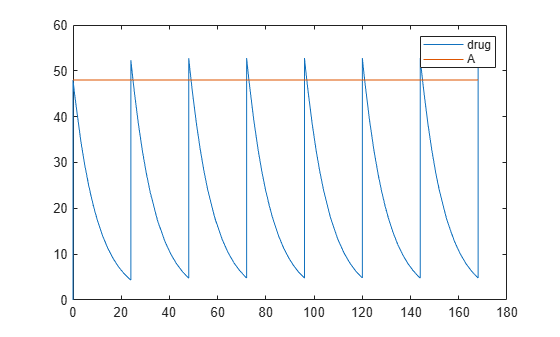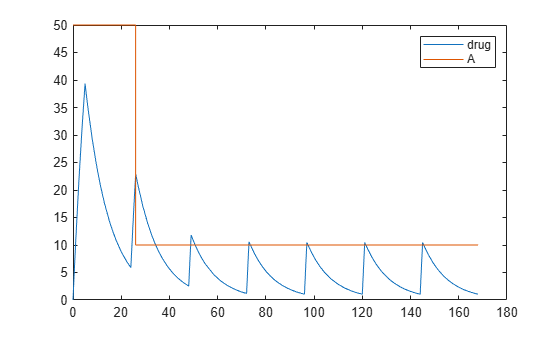RepeatDose object

Define drug dosing protocol

Description

A RepeatDose object defines a series of doses to the amount of a species during a simulation. The TargetName property of a dose object defines the species that receives the dose.

Each dose is the same amount, as defined by the Amount property, and given at equally spaced times, as defined by the Interval property. The RepeatCount property defines the number of injections in the series, excluding the initial injection. The Rate property defines how fast each dose is given.

To use a dose object in a simulation you must add the dose object to a model object and set the Active property of the dose object to true. Set the Active property to true if you always want the dose to be applied before simulating the model.

Warning

The Active property of the RepeatDose object will be removed in a future release. Explicitly specify a dose or an array of doses as an input argument when you simulate a model using sbiosimulate.

When there are multiple active RepeatDose objects on a model and if there are duplicate specifications for a property value, the last occurrence for the property value in the array of dose, is used during simulation. You can find out which dose is applied last by looking at the indices of the dose objects stored on the model.

You can set these dose properties to model parameters: Amount, Interval, Rate, RepeatCount, StartTime, LagParameterName and DurationParameterName. You can set these properties, except LagParameterName and DurationParameterName, to either a numeric value or the name of a model-scoped parameter (as a character vector or string). Parameterizing dose properties provides more flexibility for different dosing applications, such as scaling the dose amount by body weight. For details, see Parameterized and Adaptive Doses.

Constructor Summary

 sbiodose Construct dose object

Method Summary

Methods for RepeatDose objects

 copyobj Copy SimBiology object and its children delete Delete SimBiology object display Display summary of SimBiology object get Get SimBiology object properties getTable(ScheduleDose,RepeatDose) Return data from SimBiology dose object as table rename Rename object and update expressions set Set SimBiology object properties setTable(ScheduleDose,RepeatDose) Set dosing information from table to dose object

Property Summary

Properties for RepeatDose objects

 Active Indicate object in use during simulation Amount Amount of dose AmountUnits Dose amount units DurationParameterName Parameter specifying length of time to administer a dose EventMode Determine how events that change dose parameters affect in-progress dosing Interval Time between doses LagParameterName Parameter specifying time lag for dose Name Specify name of object Notes HTML text describing SimBiology object Parent Indicate parent object Rate Rate of dose RateUnits Units for dose rate RepeatCount Dose repetitions StartTime Start time for initial dose time Tag Specify label for SimBiology object TargetName Species receiving dose TimeUnits Show time units for dosing and simulation Type Display SimBiology object type UserData Specify data to associate with object

Examples

collapse all

Parameterize the Amount property of a dose to scale it by the body weight of a patient.

Create a simple model with linear elimination and an amount parameter.

model                      = sbiomodel('simple model');
compartment.CapacityUnits  = 'liter';
species.InitialAmountUnits = 'milligram';

% Elimination rate
elimParam.ValueUnits       = '1/hour';

% Elimination reaction
reaction.ReactionRate      = 'kel*drug';
amountParam.ConstantValue  = false;
amountParam.ValueUnits     = 'milligram'
amountParam =
SimBiology Parameter Array

Index:    Name:    Value:    Units:
1         A        50        milligram

Create a dose with its Amount property set to the amount parameter 'A'.

dose.Amount                = 'A';

Set other dose properties.

dose.TargetName            = 'drug';
dose.StartTime             = 0;
dose.TimeUnits             = 'hour';
dose.Interval              = 24;
dose.RepeatCount           = 7;

Add a parameter to represent the body weight.

weightParam.ValueUnits = 'kilogram';

Scale the dose amount by the body weight using an initial assignment rule.

scaleParam.ValueUnits  = 'milligram/kilogram';

Simulate the model for 7 days and plot the results.

configset               = getconfigset(model);
configset.StopTime      = 7*24;
configset.TimeUnits     = 'hour';
[time, drugAndAmount]   = sbiosimulate(model,dose);
plot(time, drugAndAmount);
legend('drug','A');Create a simple model with linear elimination, an amount parameter, and a rate parameter.

model                      = sbiomodel('simple model');
compartment.CapacityUnits  = 'liter';
species.InitialAmountUnits = 'milligram';

% Elimination rate
elimParam.ValueUnits       = '1/hour';

% Elimination reaction
reaction.ReactionRate      = 'kel*drug';

% Add amount and rate parameters
amountParam.ConstantValue  = false;
amountParam.ValueUnits     = 'milligram'
amountParam =
SimBiology Parameter Array

Index:    Name:    Value:    Units:
1         A        50        milligram

rateParam.ValueUnits       = 'milligram/hour'
rateParam =
SimBiology Parameter Array

Index:    Name:    Value:    Units:
1         R        10        milligram/hour

Create a dose with its Amount and Rate properties set to the amount and rate parameters 'A' and 'R', respectively.

dose.Amount                = 'A';
dose.Rate                  = 'R';

Set other dose properties.

dose.TargetName            = 'drug';
dose.StartTime             = 0;
dose.TimeUnits             = 'hour';
dose.Interval              = 24;
dose.RepeatCount           = 7;

Prepare the configuration set to simulate the model for 7 days.

configset           = getconfigset(model);
configset.StopTime  = 7*24;
configset.TimeUnits = 'hour';

Add an event to reset the dose amount to 10 at time >= 26.

event = addevent(model,'time >= 26','A = 10');

Set the EventMode property to 'stop'. This setting causes any ongoing dose event to stop at 26 hours.

dose.EventMode = 'stop';

Simulate the model. The second dose event stops at 26 hours, and the subsequent dose events continue with the new dose amount of 10.

[time, drugAndAmount] = sbiosimulate(model,dose);
figure
plot(time, drugAndAmount);
legend('drug','A');Alternatively, you can allow the ongoing dose event to finish before applying the new dose amount by setting EventMode to 'continue'.

dose.EventMode = 'continue';

Simulate the model. In this case, the second dose event continues to 26 hours.

[time, drugAndAmount] = sbiosimulate(model,dose);
figure
plot(time, drugAndAmount);
legend('drug','A');